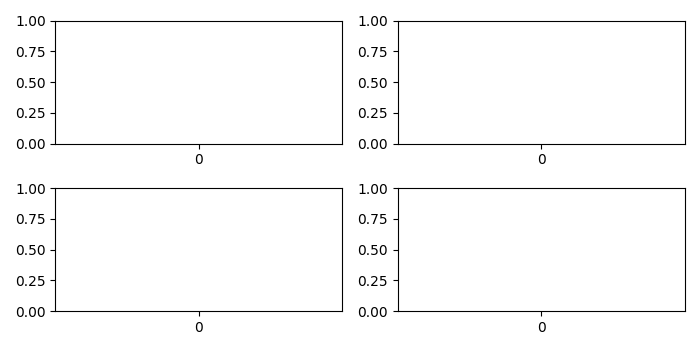# How to adjust the space between Matplotlib/Seaborn subplots for multi-plot layouts?

To adjust the space between matplotlib/seaborn subplots for multi-plot layouts, we can take the following steps

## Steps

• Set the figure size and adjust the padding between and around the subplots.

• Create a figure and a set of subplots.

• Adjust the subplot layout parameters.

• Create Seaborn's box plot for all the subplots.

• To display the figure, use Show() method.

## Example

import seaborn as sns
from matplotlib import pyplot as plt

plt.rcParams["figure.figsize"] = [7.00, 3.50]
plt.rcParams["figure.autolayout"] = True

fig, axes = plt.subplots(2, 2)

# Adjust the subplot layout parameters

# Create Seaborn boxplot for all the subplots
sns.boxplot(ax=axes[0, 0])
sns.boxplot(ax=axes[0, 1])
sns.boxplot(ax=axes[1, 0])
sns.boxplot(ax=axes[1, 1])

# Display the plot
plt.show()

## Output

It will produce the following output −